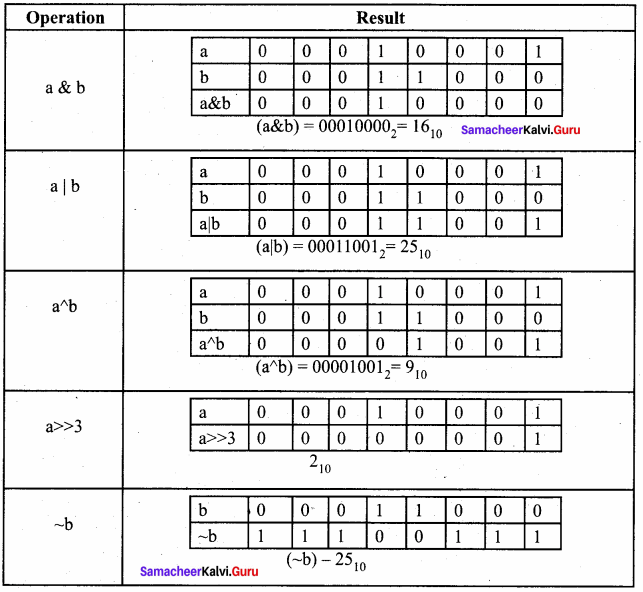Students can Download Computer Science Chapter 9 Introduction to C++ Questions and Answers, Notes Pdf, Samacheer Kalvi 11th Computer Science Book Solutions Guide Pdf helps you to revise the complete Tamilnadu State Board New Syllabus and score more marks in your examinations.

## Tamilnadu Samacheer Kalvi 11th Computer Science Solutions Chapter 9 Introduction to C++

### Samacheer Kalvi 11th Computer Science Introduction to C++ Text Book Back Questions and Answers

PART – 1

11th Computer Science Chapter 9 Book Back Answers Question 1.
Who developed C++?
(a) Charles Babbage
(b) Bjame Stroustrup
(c) Bill Gates
(d) Sundar Pichi
(b) Bjame Stroustrup

11th Computer Science Evaluate Yourself Answers Question 2.
What was the original name given to C++?
(a) CPP
(c) C with Classes
(d) Class with C
(c) C with Classes

Samacheer Kalvi Guru 11th Computer Science Question 3.
Who coined C++?
(a) Rick Mascitti
(b) Rick Bjame
(c) Bill Gates
(d) Dennis Ritchie
(a) Rick Mascitti

Samacheer Kalvi Computer Science Question 4.
The smallest individual unit in a program is:
(a) Program
(b) Algorithm
(c) Flowchart
(d) Tokens
(d) Tokens

Computer Science Samacheer Kalvi Question 5.
Which of the following operator is extraction operator of C++?
(a) >>
(b) <<
(c) <>
(d) ^^
(a) >>

Samacheer Kalvi 11th Computer Science Question 6.
Which of the following statements is not true?
(a) Keywords are the reserved words convey specific meaning to the C++ compiler.
(b) Reserved words or keywords can be used as an identifier name.
(c) An integer constant must have at least one digit without a decimal point.
(d) Exponent form of real constants consists of two parts
(b) Reserved words or keywords can be used as an identifier name.

11th Samacheer Kalvi Computer Science Question 7.
Which of the following is a valid string literal?
(a) ‘A’
(b) ‘Welcome’
(c) 1232
(d) “1232”
(d) “1232”

11th Computer Science Samacheer Kalvi Question 8.
A program written in high level language is called as ……………….
(a) Object code
(b) Source code
(c) Executable code
(d) All the above
(b) Source code

Samacheer Kalvi Computer Science Book Question 9.
Assume a = 5, b = 6; what will be result of a & b?
(a) 4
(b) 5
(c) 1
(d) 0
(a) 4

11 Computer Science Samacheer Kalvi Question 10.
Which of the following is called as compile time operators?
(a) sizeof
(b) pointer
(c) virtual
(d) this
(a) sizeof

PART – 2
II. Answer to all the questions

Samacheer Kalvi.Guru 11th Computer Science Question 1.
What is meant by a token? Name the token available in C++.
C++ program statements are constructed by many different small elelments such as commands, variables, constants and many more symbols called as operators and punctuators. Individual elements are collectively called as Lexical units or Lexical elements or Tokens. C++ has the following tokens:

1. Keywords
2. Identifiers
3. Literals
4. Operators
5. Punctuators

Samacheer Kalvi 11th Computer Science Book Back Answers Question 2.
What are keywords? Can keywords be used as identifiers?
Keywords are the reserved words which convey specific meaning to the C++ compiler. They are the essential elements to construct C++ programs. Most of the keywords are common to C, C++ and Java. Keywords are reserved and cannot be used as identifiers.

Question 3.
The following constants are of which type?

1. 39
2. 032
3. OXCAFE
4. 04.14

1. 39 – Decimal
2. 032 – Octal
4. 04.14 – Decimal

Question 4.
Write the following real constants into the exponent form:

1. 23.197 00
2. 7.214
3. 0.00005
4. 0.319

1. 23.197 = 0.23197 x 102 = 0.23197E2
2. 00 7.214 = 0.7214 x 101 = 0.7214E1
3. 0.00005 = 0.5 x 10-4 = 0.5E – 4
4. 0.319 = 3.19 x 10-1 = 3.19E – 1

Question 5.
Assume n = 10; what will be result of n>>2;?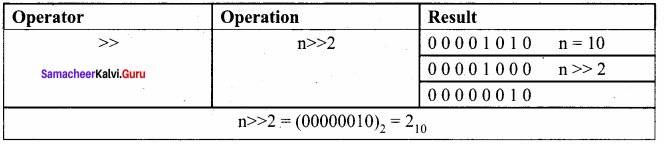Question 6.
Match the following: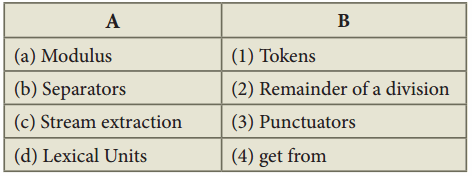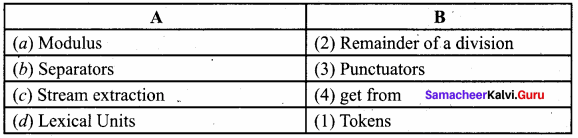PART – 3
III. Answer to all the questions

Question 1.
Describe the differences between keywords and identifiers?
Keywords:

• Keywords are the reserved words which convey specific meaning to the C++ compiler.
• They are essential elements to construct C++ programs.
• Most of the keywords are common to C, C++ and Java.

Identifiers:

• Identifiers are the user-defined names given to different parts of the C++ program.
• They are the fundamental building blocks of a program.
• Every language has specific rules for naming the identifiers.

Question 2.
Is C++ case sensitive? What is meant by the term “case sensitive”?
C++ is a case sensitive programming language so, all the keywords must be in lowercase. Case sensitive means that the uppercase and lowercase letters are considered differently.

Question 3.
Differentiate “=” and “==”.

 = = = ‘=’ is an assignment operator ‘= =’ is an equal to operator and it is a relational operator. It is used to assign the value of variable or expression It is used for comparison of both left and right side operands.

Question 4.
Assume a = 10, b = 15; What will be the value of a^b?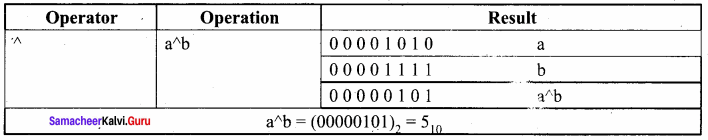Question 5.
What is the difference between “Run time error” and “Syntax error”?
Run – time Error:

• A run time error occurs during the execution of a program. It occurs because of some illegal operation that takes place.
• For example, if a program tries to open a file which does not exist, it results in a run – time error.

Syntax Error:

• Syntax errors occur when grammatical rules of C++ are violated.
• Example: if you type as follows, C++ will throw an error.
cout << “Welcome to Programming in C++”

Question 6.
What are the differences between “Logical error” and “Syntax error”?
Logical Error : Logical errors occur when there is an incorrect usage of variable / operator / order of execution etc. It is also called as Semantic Error.

Syntax Error : Syntax errors occur when grammatical rules of C++ are violated.

Question 7.
What is the use of a header file?
Header files contain definitions of Functions and Variables, which is imported or used into any C++ program by using the pre – processor #include statement. Header files have an extension “.h” which contains C++ function declaration and macro definition.
Example: #include

Question 8.
Why is main function special?
C++ program is a collection of functions. Every C++ program must have a main function. The main() function is the starting point where all C++ programs begin their execution. Therefore, the executable statements should be inside the main() function.

Question 9.
Write two advantages of using include compiler directive.

1. The program is broken down into modules, thus making it more simplified.
2. More library functions can be used, at the same time size of the program is retained.

Question 10.
Write the following in real constants.

1. 15.223
2. 211.05
3. 0.00025

1. 15.223 = 0.15223E2
2. 211.05 = 0.21105E3
3. 0.00025 = 0.25E – 3

PART – 4

Question 1.
Write about Binary operators used in C++.
Binary Operators require two operands:
Arithmetic operators that perform simple arithmetic operations like addition, subtraction, multiplication, division (+, -, *, %, /) etc. are binary operators which requires minimum of two operands.

Relational operators are used to determine the relationship between its operands. The relational operators (<, >, >=, <=, ==, !=) are applied on two operands, hence they are binary operators. AND, OR (logical operator) both are binary operators. Assignment operator is also a binary operator (+=, – =, *=, /=, %=).

Question 2.
What are the types of Errors?

 Type of Error Description Syntax Error Syntax errors occur when grammatical rules of C++ are violated. Semantic Error Semantic Error occur when there is wrong use of variable / operator / order of execution etc. It is also called as Logical Error. Run – time error A run time error occurs during the execution of a program. It is occurs because of some illegal operation that takes place.

Question 3.
Assume a = 15, b = 20; What will be the result of the following operations?
(a) a&b
(b) a|b
(c) a^b
(d) a>>3
(e) (~b)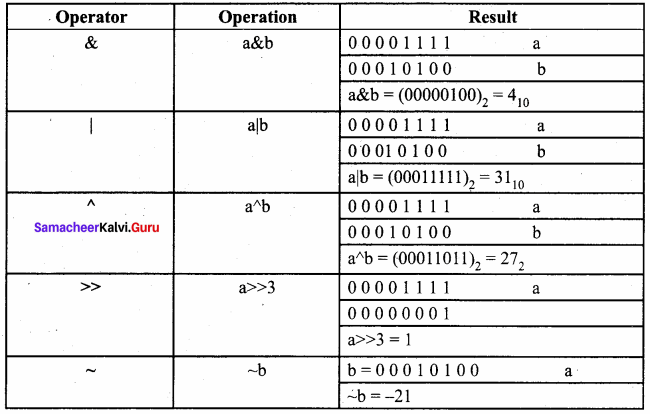PART – 1

Question 1.
How many categories of data types available in C++?
(a) 5
(b) 4
(c) 3
(d) 2
(c) 3

Question 2.
Which of the following data types is not a fundamental type?
(a) signed
(b) int
(c) float
(d) char
(a) signed

Question 3.
What will be the result of following statement?
char ch= ‘B’;
cout<< (int) ch;
(a) B
(b) b
(c) 65
(d) 66
(d) 66

Question 4.
Which of the character is used as suffix to indicate a floating point value?
(a) F
(b) C
(c) L
(d) D
(a) F

Question 5.
How many bytes of memory allocates for the following variable declaration if you are using
Dev C++? short int x;
(a) 2
(b) 4
(c) 6
(d) 8
(a) 2

Question 6.
What is the output of the following snippet?
charch =‘A’;
ch = ch + 1;
(a) B
(b) A1
(c) F
(d) 1A
(a) B

Question 7.
Which of the following is not a data type modifier?
(a) signed
(b) int
(c) long
(d) short
(b) int

Question 8.
Which of the following operator returns the size of the data type?
(a) sizeof()
(b) int ()
(c) long ()
(d) double ()
(a) sizeof()

Question 9.
Which operator is used to access reference of a variable?
(a) \$
(b) #
(c) &
(d) !
(c) &

Question 10.
This can be used as alternate to endl command:
(a) \t
(b) \b
(a) \0
(d) \n
(d) \n

PART – 2
II. Answer to all the questions

Question 1.
Write a short note const keyword with an example.
const is the keyword used to declare a constant, const keyword modifies / restricts the accessibility of a variable. So, it is known as Access modifier.

Question 2.
What is the use of setw( ) format manipulator?
setw manipulator sets the width of the field assigned for the output. The field width determines the minimum number of characters to be written in output.
Syntax:
setw(number of characters)

Question 3.
Why is char often treated as integer data type?
Character data type accepts and returns all valid ASCII characters. Character data type is often said to be an integer type, since all the characters are represented in memory by their associated ASCII Codes. If a variable is declared as char, C++ allows storing either a character or an integer value.

Question 4.
What is a reference variable? What is its use?
Reference variable in C++ is alias for existing variable. They store nothing but the address of the variable used at the time of its declaration. It is important to assign the reference variable at the time of declaration, else it will show an error.

Question 5.
Consider the following C++ statement. Are they equivalent?
char ch = 67;
charch = ‘C’;
Both the statements are equivalent as they declare ‘ch’ to be char and initialize it with the value of 67. Since this is the ASCII code for ‘C’, the character constant also can be used to initialize ‘ch’ to 67.

Question 6.
What is the difference between 56L and 56?
56L – The suffix L forces the constant to be represented as long, which occupies 4 bytes.

56 – This will be represented as int type constant which occupies 2 bytes as per Turbo C++.

Question 7.
Determine which of the following are valid constant? And specify their type.

1. 0 0.5
2. ‘Name’
3. ‘\t’
4. 27,822

1. 0.5 – is a valid constant. It is a decimal.
2. ‘Name’ – Invalid constant as single quote is not allowed.
3. ‘\t’ – Escape sequence (or) non – graphical character (horizontal tab).
4. 27,822 – Invalid constant. Comma is not allowed.

Question 8.
Suppose x and y are two double type variable that you want add as integer and assign to an integer variable. Construct a C++ statement for the doing so.
double x = 10.5, y = 4.5; int a;
a = int (x) + int (y);

Question 9.
What will be the result of following if num=6 initially.
(a) cout << num;
(b) cout << (num==5);
(a) 6 (b) False

Question 10.
Which of the following two statements are valid? Why? Also w rite their result, int a; a = 3,014; a=(3,014);
It is invalid as comma is not allowed in an integer constant. It is valid. Comma in bracket is allowed.

PART – 3
III. Answer to all the questions

Question 1.
What are arithmetic operators in C++? Differentiate unary and binary arithmetic operators. Give example for each of them.
Arithmetic operators : perform simple arithmetic operations like addition, subtraction, multiplication, division etc.
Unary Operators : Require only one operand . Example: +, -, *, /, %, >, <, <=, AND, OR

Binary Operators:

1. Require two operands
2. Example: ++ (Plus, Plus) Increment operator, – – (Minus, Minus) Decrement operator, NOT, ~,

Question 2.
Evaluate x + = x + + + x; Let x = 5;
x + = x + + + x (x = 5) x + = x + + + 5 (x becomes 6)
x + = 6 + 6
x + = 12
x = 6 + 12
x = 18

Question 3.
How relational operators and logical operators are related to one another?
Relational operators are used to determine the relationship between its operands. When the relational operators are applied on two operands, the result will be a boolean value 1 or 0 which represents True or False respectively which represents logical operator.

Question 4.
Evaluate the following C++ expressions where x, y, z are integers and m, n are floating point numbers. The value of x = 5, y = 4 and m = 2.5;

1. n = x + y / x;
2. z = m * x + y;
3. z = (x++) * m + x;

1. n = x + y / x;
= 5 + 4/5
= 5 + 0 (both x and y are int type. Therefore only integer part of quotient is considered)
=5

2. z = m * x + y;
= 2.5 * 5 + 4 (m is float type, so x value is promoted to float [implicit conversion])
= 12.5 + 4 ‘
= 16 (2 is int type. So ‘.2’, the fractional part is discarded)

3. z = (x++) * m + x;
= 5*2.5 + x
= 12.5 + 5
= 18 (z is int type, therefore the fractional part is removed, x is incremented after the addition)

### Samacheer kalvi 11th Computer Science Introduction to C++ Additional Questions and Answers

PART – 1

Question 1.
The latest standard version published in December 2017 as ISO/IEC …………….. which is informally known as C++ 17.
(a) 14882 : 1998
(b) 14883 : 2017
(c) 14882 : 2017
(d) 14882 : 2000
(c) 14882 : 2017

Question 2.
The smallest individual unit in a program is known as ……………..
(a) token
(b) lexical unit
(c) lexical element
(d) all the above
(d) all the above

Question 3.
Integer constant is also called as ……………..
(a) fixed point constant
(b) floating point constant
(c) real constants
(d) boolean literals
(a) fixed point constant

Question 4.
Exponent form of real constants consists of parts.
(a) 3
(b) 2
(c) 5
(d) 4
(b) 2

Question 5.
…………….. relational operators are binary operators.
(a) 7
(b) 8
(c) 6
(d) 2
(c) 6

Question 6.
Match the following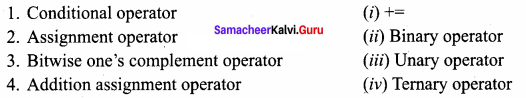(a) 1 – (i) 2 – (ii) 3 – (iii) 4 – (iv)
(b) 1 – (iv) 2 – (ii) 3 – (iii) 4 – (i)
(c) 1 – (i) 2 – (iii) 3 – (ii) 4 – (iv)
(d) 1 – (i) 2 – (ii) 3 – (iv) 4 – (iii)
(b) 1 – (iv) 2 – (ii) 3 – (iii) 4 – (i)

Question 7
…………….. used to label a statement.
(a) colon
(b) comma
(c) semi – colon
(d) parenthesis
(a) colon

Question 8.
Match the following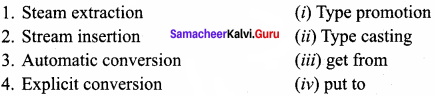(a) 1 – (i) 2 – (ii) 3 – (iii) 4 – (iv)
(b) 1 – (ii) 2 – (iv) 3 – (i) 4 – (iii)
(c) 1 – (i) 2 – (iii) 3 – (ii) 4 – (iv)
(d) 1 – (i) 2 – (ii) 3 – (iv) 4 – (iii)
(d) 1 – (i) 2 – (ii) 3 – (iv) 4 – (iii)

Question 9.
IDE stands for ……………..
(a) Integrated Development Environment
(b) International Development Environment
(c) Integrated Digital Environment
(d) None of the above
(a) Integrated Development Environment

Question 10.
In programming language …………….. are referred as variables and the values are referred to as data.
(a) constant
(b) integer
(c) fields
(d) files
(c) fields

Question 11.
…………….. data type signed more precision fractional value.
(a) char
(b) short
(c) long double
(d) signed doubles
(c) long double

Question 12.
Syntax for reference is ……………..
(a) =
(b) = <& reference>
(c) <&reference_variable>=
(d) None of these
(c) <&reference_variable>=

Question 13.
…………….. manipulator is the member of iomanip header file.
(a) setw
(b) setfill
(c) setf
(d) all the above
(d) all the above

Question 14.
…………….. is used to set the number of decimal places to be displayed.
(a) Set precision
(b) Garbage
(c) Constant
(d) All the above
(a) Set precision

Question 15.
Match the following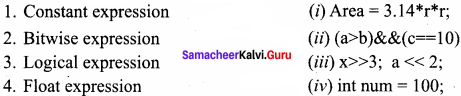(a) 1 – (iv) 2 – (iii) 3 – (ii) 4 – (i)
(b) 1 – (i) 2 – (ii) 3 – (iii) 4 – (iv)
(c) 1 – (iv) 2 – (i) 3 – (i) 4 – (iii)
(d) 1 – (iv) 2 – (iii) 3 – (i) 4 – (ii)
(a) 1 – (iv) 2 – (iii) 3 – (ii) 4 – (i)

PART – 2

Question 1.
Mention any two benefits of C++.

1. C++ is a highly portable language and is often the language of choice for multi – device, multi – platform app development.
2. C++ is an object – oriented programming language and includes classes, inheritance, polymorphism, data abstraction and encapsulation.

Question 2.
What is the use of Boolean literals?
Boolean literals are used to represent one of the Boolean values (True or false). Internally true has value 1 and false has value 0.

Question 3.
What are the types of C++ operators based on the number of operands?
The types of C++ operators based on the number of operands are:

1. Unary Operators – Require only one operand
2. Binary Operators – Require two operands
3. Ternary Operators – Require three operands

Question 4.
What are the types of bitwise operators?
In C++, there are three kinds of bitwise operators, which are:

1. Logical bitwise operators
2. Bitwise shift operators
3. One’s compliment operators

Question 5.
What is stream extraction operator?
C++ provides the operator >> to get input. It extracts the value through the keyboard and assigns it to the variable on its right; hence, it is called as “Stream extraction” or “get from” operator.

Question 6.
Expand IDE and GNU.
IDE stands for Integrated Development Environment and GNU stands for General Public License.

Question 7.
What are the main types of C++ datatypes?
In C++, the data types are classified as three main categories

1. Fundamental data types
2. User – defined data types and
3. Derived data types.

Question 8.
Write C++ program to accept any character and display its next character.
C++ Program to accept any character and display its next character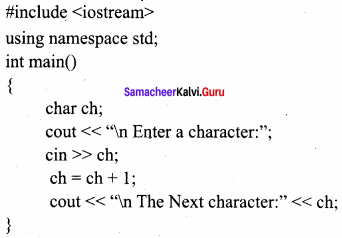The output produced by the program will be
Enter a character: A
The Next character: B

Question 9.
Write C++ program to find the area of circle.
C++Program to find the area of circle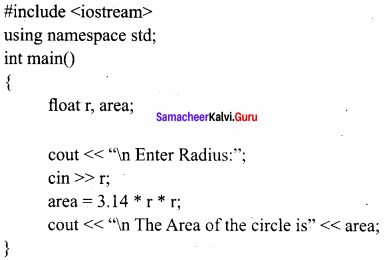Output:
The Area of the circle is 132.665

Question 10.
What is Junk (or) Garbage values?
If you declare a variable without any initial value, the memory space allocated to that variable will be occupied with some unknown value. These unknown values are called as “Junk” or “Garbage” values.

PART – 3
III. Explain in Brief

Question 1.
What are the uses of Set precision?
setprecision () prints the values from left to right. For the above code, first, it will take 4 digits and then prints one digit from fractional portion. setprecision can also be used to set the number of decimal places to be displayed. In order to do this task, you will have to set an ios flag within setf() manipulator. This may be used in two forms: fixed and (i) fixed (ii) scientific These two forms are used when the keywords fixed or scientific are appropriately used before the setprecision manipulator.

Question 2.
Define – Expression?
An expression is a combination of operators, constants and variables arranged as per the rules of C++. It may also include function calls which return values.

Question 3.
What is Automatic conversion and Type promotion?
An Implicit type conversion is a conversion performed by the compiler automatically. So, implicit conversion is also called as “Automatic conversion”. This type of conversion is applied usually whenever different data types are intermixed in an expression. If the type of the operands differ, the compiler converts one of them to match with the other, using the rule that the “smaller” type is converted to the “wider” type, which is called as “Type Promotion”.

Question 4.
What is type casting. Write its syntax?
C++ allows explicit conversion of variables or expressions from one data type to another specific data type by the programmer. It is called as “type casting”.
Syntax:
(type – name) expression;
Where type – name is a valid C++ data type to which the conversion is to be performed.

Question 5.
What kind of constants are the following?

1. 0X568
2. – 27
3. – 27

2. Decimal
3. Octal

Question 6.
What are the types of C++operators?
C++ Operators are classified as:

1. Arithmetic Operators
2. Relational Operators
3. Logical Operators
4. Bitwise Operators
5. Assignment Operators
6. Conditional Operator
7. Other Operators

Question 7.
Convert the following real constants into exponent form:

1. 12.0005
2. 0.00000009
3. 7.9283

0 12.0005 = 0.120005 x 102 = 0.120005E2
0.00000009 = 0.9 x 10-7 = 0.9E-7
7.9283 = 0.79283 x 101 = 0.79283E1

Question 8.
If a = 15; what is the result of a<<3 and a >> 2?
If a = 15; equivalent binary value of a is 00001111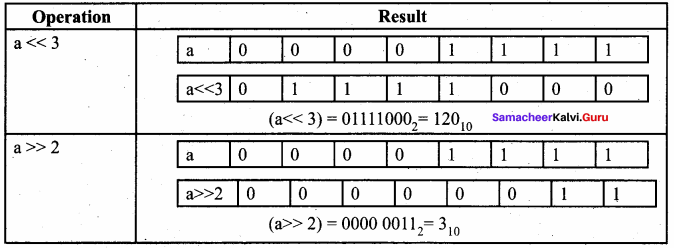Question 9.
If b = 15 what is the result of ~b?
If b = 15; equivalent binary value of b is 00001111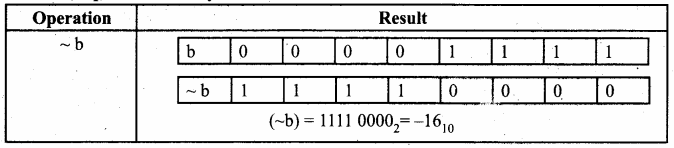Question 10.
If a = 65, b = 15, what is the result of a|b?
Equivalent Binary values of 65 = 01000001; 15 = 00001111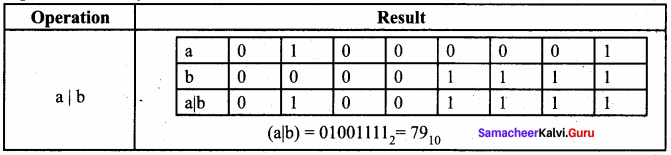PART – 4
IV. Explain in Detail

Question 1.
Explain steps involved in creating and execution of C++ program.
For creating and executing a C++ program, one must follow four important steps.
1. Creating Source code : Creating includes typing and editing the valid C++ code as per the rules followed by the C++ Compiler.

2. Saving source code with extension .cpp
After typing, the source code should be saved with the extension .cpp

3. Compilation
This is an important step in constructing a program. In compilation, compiler links the library files with the source code and verifies each and every line of code. If any mistake or error is found, it will inform you to make corrections. If there are no errors, it translates the source code into machine readable object file with an extension .obj

4. Execution
Execution is the final step of construction of a C++ Program. In this stage, the object file becomes an executable file with extension .exe. Once the program becomes an executable file, the program has an independent existence. This means, you can run your application without the help of any compiler or IDE.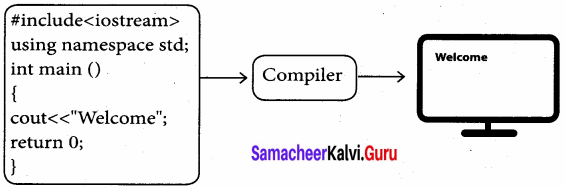Question 2.
Explain working with Dev C++.
Dev C++ is an open source, cross platform (alpha version available for Linux), full featured Integrated Development Environment (IDE) distributed with the GNU General Public License for programming in C and C++. It is written in Delphi. It can be downloaded from
http://www.bloodshed.net/dev/devcpp.html

After installation Dev C++ icon is available on the desktop. Double click to open IDE. Dev C++ IDE appears.
To create a source file, Select File → New → Source file or Press Ctrl + N.
On the screen that appears, type your C++ program, and save the file by clicking File → Save or Pressing Ctrl + S. It will add .cpp by default at the end of your source code file. No need to type .cpp along with your file name.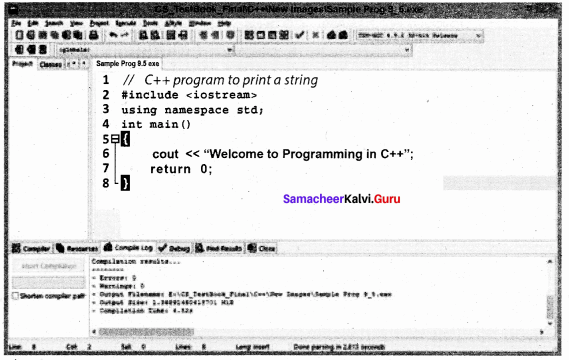After save, Click Execute → Compile and Run or press F11 key.
After successful compilation, output will appear in output console, as follows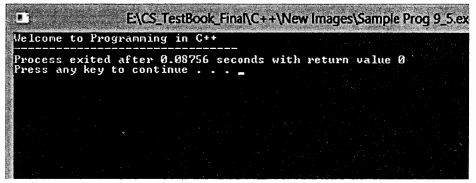Question 3.
Draw memory allocation of a variable and int type variable.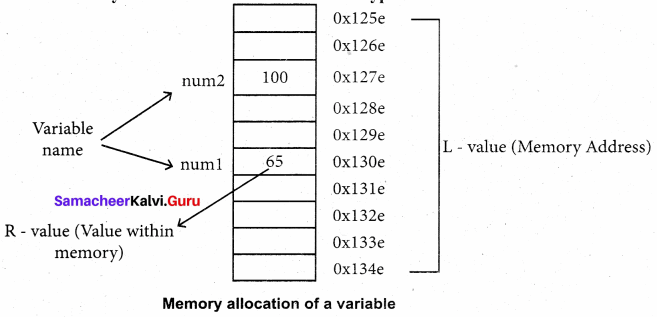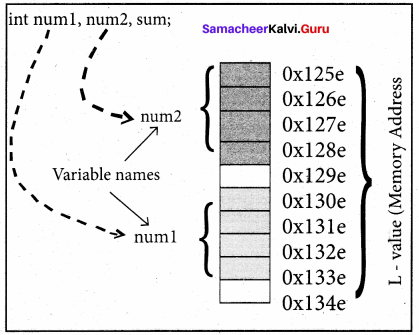Question 4.
Write the C++ program to find the curved surface area of the cylinder.
C++ Program to find the Curved Surface Area of a cylinder (CSA) (CSA = 2 nr * h)
#include
using namespace std;
int main()
{

float pi = 3.14, radius, height, CSA;
cout << “\n Curved Surface Area of a cylinder”;
cout << “\n Enter Height (in cm):”; cin >> height;
cout << “\n Height:” << height« “cm”;
cout << “\n Curved Surface Area of a Cylinder is” << CSA «“sq. cm.”;

}

Output:
Curved Surface Area of a cylinder
Enter Radius (in cm) : 7
Enter Height (in cm) : 20
Height : 20cm
Curved Surface Area of a Cylinder is 879.2 sq. cm.

Question 5.
Write the C++ program to calculate Net salary.
Program to Calculate Net Salary
#include
#include using namespace std;
int main()
{

float basic, da, hra, gpf, tax, gross, np; char name;
cout << “\n Enter Basic Pay:”; cin >> basic;
cout<< “\n Enter D.A cin >> da;
cout << “\n Enter H.R.A:”; cin >> hra;
gross = basic+da+hra; // sum of basic, da nad hra
gpf = (basic+da) * 0.10; //10% of basic and da
tax = gross * 0.10; //10% of gross pay
np = gross – (gpf+tax); //netpay = earnings – deductions
cout << setw(25) << “Basic Pay:” << setw(10) << basic << endl;
cout << setw(25) << “Dearness Allowance:”<< setw(10) << da << endl;
cout << setw(25) << “House Rent Allowance:” << setw(10) << hra << endl;
cout << setw(25) << “Gross Pay:” << setw(10) << gross << endl;
cout << setw(25) << “G.P.F:” << setw(10) << gpf << endl;
cout << setw(25) << “Income Tax:” << setw(10) << tax << endl;
cout << setw(25) << “Net Pay:” << setw(10) << np << endl;

}
The output will be,
Enter Basic Pay : 12000
Enter D.A : 1250
Enter H.R.A : 1450
Basic Pay : 12000
Dearness Allowance : 1250
House Rent Allowance : 1450
Gross Pay : 14700
G.P.F : 1325
Income Tax : 1470
Net Pay : 11905

Question 6.
If a = 17, b = 24, what is the result of the following?

1. a & b
2. a|b
3. a^b
4. a>>3
5. ~ b